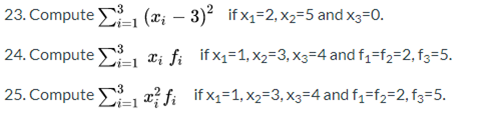## MA 171 Week 7 Discussion | Park University | Assignment Help

MA 171 Week 7 Discussion | Park University | Assignment Help

Week 7 Discussion

1. Why is it important to represent data with graphing tools?

2. What are the similarities and differences between a histogram and a frequency table?

3. What is the difference between a frequency polygon and a cumulative frequency polygon? Explain.

4. In a given frequency table, if  represents the total sum of the frequencies and if  denotes a frequency of a class, then what is the relative frequency of that class in terms of  and ? Explain.

5. What is the difference between the relative frequency and the cumulative relative frequency?

6. In constructing the ogive, do we need to know what is the frequency of each class, or knowing the cumulative for each class is sufficient? Explain.

7. A given frequency table has 3 classes, Class1, Class2, and Class3. The total number of frequencies is 10 and Class1 and Class2 have frequencies 2 and 3 respectively. What is the relative frequency of Class3?

8. Compute the mean of the following data: 4, 3, 6, 6, 6. Explain your work.

9. Given the following data: 3, 3, 4, 5, 7, 7, 7. What is the mode, median, and the mean? Explain.

10. A fair die is rolled 300 times and each time the result is recorded. What would you expect the mean of the formed data set be? Explain.

11. A data set is formed by recording  the sums on a 100 rolls of a pair of a fair dice. What would you expect the mean of the data set be?

12. Construct a set of four numbers that have mean 10, mode 8, and median 9. Explain.

13. Suppose we have a data of size two, say x1 and x2 whose mean is 5. If x1=3, compute the value of x2.

14. Suppose that we have the data {x1, x2, ...,xn} such that x1=x2=...=xn=3. What is the mean of the data? What is the standard deviation?

15. In the formula for the mean of the grouped data, what do you get when you have all group frequencies equal to 1?

16. Compute the standard deviation of the following data: 3, 3, 4, 6.

17. Suppose we have a data of size two, say x1 and x2. What is the standard deviation?

18. Explain why the variance is never negative.

19. Suppose we have a data whose standard deviation is zero. What conclusion can you derive about the data?

20. Two data sets of the same size have standard deviations s1 and s2. If s1<s2, then how do these data sets compare?

21. A data set is formed by recording the sums in 200 rolls of a pair of a dice. A second data set is formed by recording the results of 200 draws of a ball from a box containing 11 balls numbered 2 through 12. Which of the data sets would you expect to have a smaller standard deviation? Explain.

22. Assume that we have a data of size 3 with mean 5 and mode 3. Compute the standard deviation.